I.2.10c

2/16/2021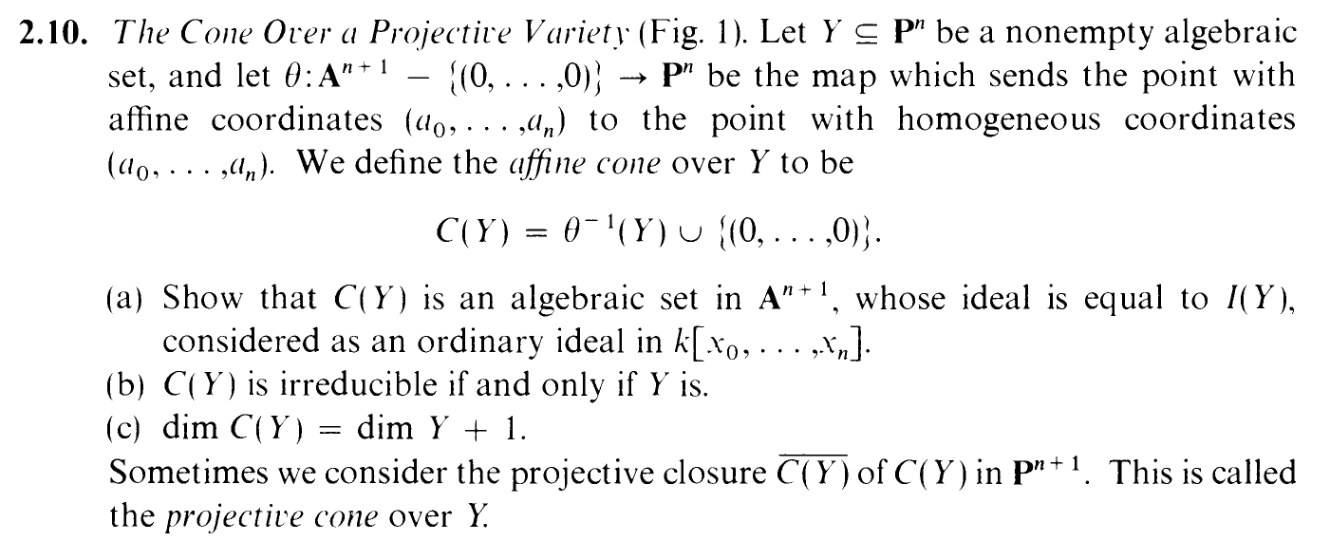Well, somebody had a tantrum yesterday. I wonder who that might be? What? Me, you say? Heh. "FUCJOISAHFOIAFHWQOIRH329MC932QV90832QNMY90832NY5CM3209RMY32Q42985CMNY32980C32QY0932NMY5CY3252Y0NYC932NNCM0YNM01NMY0XY3NNR9NMQECYRW8NR3Q9NCR2930NYC0932QNCR932QNYRC09732Q9RNYC3982QNYCR032NC97R32NYC092QNYRC0932CR932YCN932QNYC02QNYC0932QNYRC9732NYC209QNYCR02QNYC0R2QNYCR093Q2NYV09CY032NCRY02QNC032NC03CN3N093NCQ90NC0973QNYCQN523C" is actually my calmness incantation–an advanced holeinmyheart koan I use to assemble brain power when I'm about to plunge into a productive work session. It's called the the pomoFUCJ technique. Try it some time.

Now, the tempting thing for (c) is to apply 2.6, but HOLD IT! 2.6 assumes we're working with a variety (irreducible), whereas here we can only assume that our guy is algebraic (closed). Oof.

Hmmmm. Well... if irreducible sets are more convenient, we can break up Y into irreducible sets, thanks to 2.5: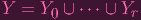(1)

And note that dim Y = sup dim Y i (Cause if C1 C2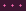Cd is a chain of irreducible closeds in Y , then Cd has to be contained in one of the Y i's, so the Y i's will always be the tippy top of any chains). Assume without loss of generality that sup dim Y i = dim Y 0, so dim Y = dim Y 0

Now since Y i is irreducible, we can use 2.6. Also remember the corresponding proposition for affines: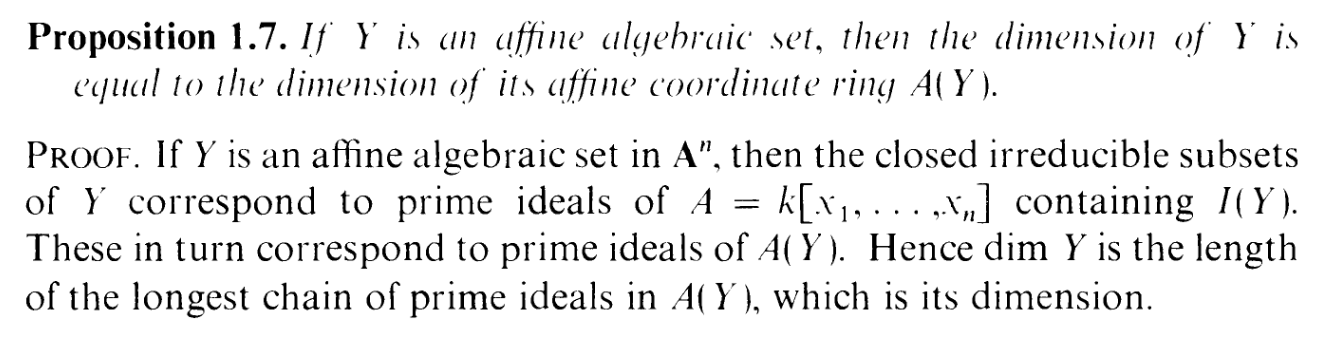dim Y 0 = dim S(Y 0) - 1 (by 2.6) = dim k[x0,…,xn]∕I(Y 0) - 1 (definition of S(⋅)) = dim k[x0,…,xn]∕I(C(Y 0)) - 1 (part (a)) = dim A(C(Y 0)) - 1 (definition of A(⋅)) = dim C(Y 0) - 1 (prop 1.7)

What I essentially just showed was that if we are working with a projective variety (i.e. irreducible closed set) X, then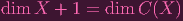(2)

(so I basically proved this exercise for the "easy" case)
But remember! dim Y = dim Y 0, so we can actually say that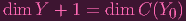(3)

Errrrr..... Fuck. That's supposed to be C(Y ) on the other side, not C(Y 0). Yeah.... C(Y 0) is merely a subset of C(Y ). But perhaps we can use that same trick we did for Y , where we break C(Y ) into irreducible components, using the affine correspondent of 2.5: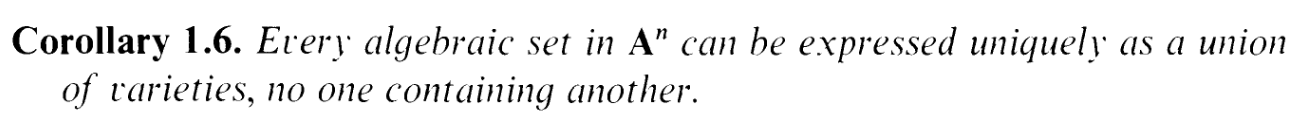and conclude that dim C(Y ) = dim C(Y 0).... But is C(Y 0) even an irreducible component? And does it have the highest dimension among the irreducible components?

I know that C(Y 0) is irreducible, at least, thanks to part (b), but I haven't shown that it's an irreducible component (i.e. maximal).

It would be nice if C(Y 0) were an irreducible component of Y . Is it?

Well, I spent like 2 hours trying to show this. I let V be an irreducible component where C(Y 0) V Y and tried to show that V = C(Y 0) (hence making C(Y 0) maximal), but that... did not turn out so well. I won't bother you with the details (okay I will. can't miss a HOLEINMYHEART ADMIN FUCKUP SESSION (tho if youre feeling h0rny, meet me after this post for a holeinmyheart fuck session): I took I(C(Y 0)) I(V ) I(Y )I(C(Y 0))Sh I(V )Sh I(C(Y 0))Sh, taking Z in projective space, Z(I(C(Y 0) Sh) Z(I(V ) Sh) Z(I(C(Y )) Sh)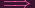Z(I(C(Y 0)) Z(I(V ) Sh) Z(I(C(Y )) by definition of I() on homogenous ideals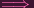Z(I(Y 0)) Z(I(V ) Sh) Z(I(Y )) thanks to part (a)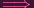Y 0 Z(I(V ) Sh) Y and from here, having escaped parenthesis hell, I tried to make some contradiction involving the middle guy needing to be reducible, so I threw everything back into affines, which, of course, put me back in parenthesis hell, as well as not really being helpful in general. FUCKUP SESSION END. "Actually, your fuckup session is your whole life". OHHHHHHHHH).

So I was thinking "hmmmm maybe C(Y 0) isn't an irreducible component of Y after all. But for some reason I had a little lightbulb moment: Let me not consider C(Y 0) by itself, but C(Y 0),C(Y 1),,C(Y r) altogether.

Take a look at Corollary 1.6 again:*AHEM* "uniquely" *AHEM* "no one containing the other".

Let me make up a little lemma to help me:

 C(A ∪ B) = θ-1(A ∪ B) ∪ 0 = θ-1(A) ∪ θ-1(B) ∪ 0 = θ-1(A) ∪ θ-1(B) ∪ 0 ∪ 0 = (θ-1(A) ∪ 0) ∪ (θ-1(B) ∪ 0) = C(A) ∪ C(B)

so(4)

And here's another lemma:

 C(A) ⊂ C(B)θ-1(A) ∪ 0 ⊂ θ-1(B) ∪ 0θ-1(A) ⊂ θ-1(B)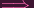θ(θ-1(A)) ⊂ θ(θ-1(B))A ⊂ B (see the beginning of last post)

So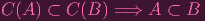(5)

Hence

 C(Y ) = C(Y 0 ∪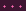∪ Y l) = C(Y 0) ∪∪ C(Y l)

Now, I already know each of these C(Y i) are irreducible, from (b). And since their union forms the entirety of C(Y ) ((4)), and none of them contain each other (else (5) yieldsa contradiction), then these have to be the irreducible components of C(Y ), by uniqueness. PHEW.

Finally, thanks to (2), C(Y 0) has to have the maximum degree of the components if Y 0 does.
So let's finish this off
 dim C(Y ) = sup dim C(Y i) = dim C(Y 0)
And combining this with (3) wraps it up...

And there's that. Finally this 3-parter is done and we can go to 2.11... which is also a 3-parter. NOOOOOOOOOOOOOOOOOOOOOOOOOOOOOOOOO.